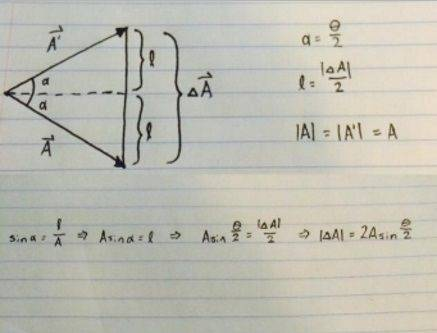# (Kleppner/Kolenkow) Missing Diagram?

• Cosmophile

#### Cosmophile

Hello! I'm working my way through K&K (First Edition) and have encountered a slight dilemma. On page 26, I believe there may be a missing diagram. There is a reference made to a diagram which reads:

Using the angle Δθ defined in the sketch,

$$\left |\Delta A \right |=2A sin \frac{\Delta \theta}{2}$$

Unfortunately, I do not see the sketch they are referring to, and there is quite a large blank space on the page between the first diagram and the other two. I'd really hate to miss out on anything in this first chapter, as it seems to really be establishing the fundamental understandings I'll need going forward. Any help here is greatly appreciated.

Mind you all, my geometry isn't too great; I imagine I could probably derive an appropriate diagram myself if I had more experience with Geometry.

Update: I sat back down and almost immediately realized what the diagram should look like. Hooray!

I am having precisely the same issue, though I am not seeing exactly what the diagram should look like. I am having trouble seeing where the ## 2A ## and the ## \frac{\Delta \theta}{2} ## specifically are coming from. I seemed to easily be able to do the same problem by using a diagram representing a triangle formed by the vectors ## \Delta \boldsymbol A = \boldsymbol A(t + \Delta t) - \boldsymbol A(t) ##, with ## \Delta \theta ## as the angle between ## \boldsymbol A(t) ## and ## \boldsymbol A(t + \Delta t) ##. Would you be able to explain where the factors of 2 are coming into play?

I had to dig around for it, but here is what I came up with (I edited the picture to include the diagram and the equations):In case you can't read the text at the bottom:

$$\sin \alpha = \frac {l}{A} \Longrightarrow A \sin \alpha = l$$

$$\Longrightarrow A \sin \frac {\theta}{2} = \frac {|\Delta A|}{2} \to |\Delta A| = 2A \sin \frac {\theta}{2}$$

However, it is important to note that ##\Delta A## is actually the length of the arc (not shown in my diagram) and not the length shown here. That was a mistake on my part.

Alrighty, so it seems that it is largely in just the way that the diagram is made. The diagram that I constructed was pretty much the same, save the fact that I only dealt with the angle ## \theta ##. It seems to be valid either way. Thank you so much for your help! I was worried that there was something I was completely missing.

Sure thing. I'm glad I could help. In case you, or anyone else who stumbles upon this, I think this may be a better way of depicting the situation:

If my diagram was drawn in such a way that ##\Delta A## was the length of the arc, and recalling that the length of an arc ##S = r \theta## we would have
$$S = r \theta \Longrightarrow = 2|A| \frac {\Delta \theta}{2} \Longrightarrow \Delta A = |A| \Delta \theta$$

The ##2A \sin \frac {\Delta \theta}{2}## is necessary, as they reference the fact that for ##\Delta \theta \ll 1, \sin \frac {\Delta \theta}{2} \simeq \frac {\Delta \theta}{2}##.